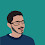# Chi-Square : Variable Reduction Technique

Chi-Square as variable selection / reduction technique

The Pearson / Wald / Score Chi-Square Test can be used to test the association between the independent variables and the dependent variable.

A Wald/Score chi-square test can be used for continuous and categorical variables. Whereas, Pearson chi-square is used for categorical variables. The p-value indicates whether a coefficient is significantly different from zero.

Note : Wald and Score Chi-Square tests are asymptotically equivalent.

For Continuous variables

Run UNIVARIATE Score / Wald Chi-Square analysis on each of your continuous independent variable with dependent variable. It means putting one variable in independent variable list and setting your dependent variable and run regression analysis. Score and Wald Chi-Square are asymptotically equivalent. In PROC LOGISTIC, use options: selection=stepwise maxstep=1 details

MAXSTEP=1 means that the maximum number of times any of the independent variables can be added or removed is 1 time.
Look at table - "Analysis of Effects Eligible for Entry"
It helps to know the univariate predictive power of each continuous variable.

Rule :
1. Keep all the variables with a probability of chi-square of less than 0.25.
2. Select the 50 variables with the highest chi-square score.
ODS OUTPUT EFFECTNOTINMODEL = TEST;
PROC LOGISTIC DATA = BHALLA.MODELING DESC;
MODEL ATTRITION = DDA DDABAL DEP DEPAMT
/ SELECTION = S MAXSTEP = 1 DETAILS;
RUN;

For Categorical variables

Run UNIVARIATE Pearson Chi-Square analysis on each of your categorical independent variable with dependent variable.  In PROC FREQ , use options: missing chisq

proc freq data= newdata;
tables attrition * balance/missing ChiSq;
run;
Keep categorical variables where each category has more than about 25 observations and a p-value <=0.25.

For Transformed Variables (Log, sqrt, exp, cube root, inverse etc.)

In PROC LOGISTIC, use options : selection=stepwise maxstep=2 details
Look at "Summary of Stepwise Procedure" table. It will give you two best variables.
Use command ODS OUTPUT ModelBuildingSummary = TEST; to store the 'Summary of Stepwise Procedure' table in SAS

Note : Use of conservative sig level (0.05) often leads to important variables excluded from analysis. It is recommended to use higher level based on the work by Mickey and Greenland (1989) on logistic regression. It is important to CHECK the IMPORTANCE of these variables in multivariable model stage before deciding your final variables.
Related Posts
ShareDeepanshu founded ListenData with a simple objective - Make analytics easy to understand and follow. He has over 10 years of experience in data science. During his tenure, he worked with global clients in various domains like Banking, Insurance, Private Equity, Telecom and HR.

8 Responses to "Chi-Square : Variable Reduction Technique"
1.Why 0.25 and not 0.05?

2.Use of conservative sig level (0.05) often leads to important variables excluded from analysis. It is recommended to use higher level based on the work by Mickey and Greenland (1989) on logistic regression. It is important to CHECK the IMPORTANCE of these variables in multivariable model stage before deciding your final variables.

3.ok u mean running the logistic regression with no selection options and viewing p-values of each variable before seeting p-value...but not more than 0.25?

1.Step 1 : Run logistic regression on each of the independent variable and selecting all the variables having p-value less than 0.25. For example, you have 10 independent variables, so run UNIVARIATE logistic regression 10 times for each of the variable and recording their p-values.

Step 2 : Run multivariate logistic regression on all the variables selecting at step 1 and now set p-value 0.05.

4.Pls i posted questions under the following topics: model validation, scoring in logistic, bootstrapping.

tnx

1.I would request you to post your question as an identified user. I don't want to encourage readers post comments as "Anonymous". Thanks!

2.Ok. Changd now. Thanks for that. I guess the same process goes for categorical variables using proc freq?

5.Hi Sir, do you have another chapter which deals with feature selection of categorical variable using chi square test in R?

Next → ← Prev
Looks like you are using an ad blocker!

To continue reading you need to turnoff adblocker and refresh the page. We rely on advertising to help fund our site. Please whitelist us if you enjoy our content.